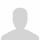# Welcome!

This community is for professionals and enthusiasts of our products and services.
Share and discuss the best content and new marketing ideas, build your professional profile and become a better marketer together.

# Overtime Computation per Overtime Code

Using this module we purchased: https://apps.odoo.com/apps/modules/12.0/to_hr_overtime_payroll/

I want to create a different salary rule with different computation base on Overtime HOUR TOTAL for each Overtime Type group by type. How would I achieve it using python code?

For Example I have OTREG and OTSUN

CODE : OTHOUR : SALARY RULE : PYTHON CODE

OTREG0006 : 05 : REGULAR OVERTIME : ????

OTREG0617 : 05 : REGULAR OVERTIME : ????

OTSUN0006 : 08 : SUNDAY OVERTIME : ????

OTSUN0617 : 04 : SUNDAY OVERTIME : ????You can filter the ot lines by the code of the desired rate. For example, below is the code for the OTREG0006

```amount = 0.0
for line in payslip.payslip_ot_line_ids.filtered(lambda l: l.overtime_rule_id.code == 'OTREG0006'):
amount += 20 * line.number_of_hours * line.rate / 100.0
result = amount
```actually, the computation is right, but i want to separate all each overtime rate into different salary rule computation for intensive report purpose.There is a rule named "Overtime Allowance Amount" added by this module, in which the computation is as follow

```# find total working hours
total_hours = sum(line.number_of_hours for line in payslip.worked_days_line_ids)

# cost per hour is calculated base on contract's wage and above found total hours
hour_cost = total_hours and contract.wage / total_hours or 0.0

# start calculating overtime cost
amount = 0.0
for line in payslip.payslip_ot_line_ids:
if payslip.contract_id:
amount += hour_cost * line.number_of_hours * line.rate / 100
result = amount
```

As for your case, I will need more information on how you caculate your overtime. I.e.: base amount, rate for each type of overtime against the base.

I assume that
• base amount: \$20/hour
• rate of the OTREG0006: 150%
• rate of the OTREG0617: 150%
• rate of the OTSUN0006: 200%
• rate of the OTSUN0617: 200%
• the employee has 10 overtime hours against the OTREG0006 and 5 overtime hours against the OTSUN0006 and 7 overtime hours against the OTSUN0617 and

The python code below could give you the result as 780
```amount = 0.0
for line in payslip.payslip_ot_line_ids:
amount += 20 * line.number_of_hours * line.rate / 100.0
# after the loop, the cummulative amount should be 780.0
result = amount
```